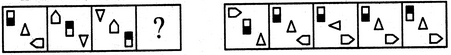# Non Verbal Reasoning - Analogy - Discussion

### Discussion :: Analogy - Section 1 (Q.No.29)

Each of the following questions consists of two sets of figures. Figures A, B, C and D constitute the Problem Set while figures 1, 2, 3, 4 and 5 constitute the Answer Set. There is a definite relationship between figures A and B. Establish a similar relationship between figures C and D by selecting a suitable figure from the Answer Set that would replace the question mark (?) in fig. (D).

29.

Select a suitable figure from the Answer Figures that would replace the question mark (?).

Problem Figures:                            Answer Figures:(A)     (B)      (C)     (D)                  (1)      (2)      (3)      (4)      (5)

 [A]. 1 [B]. 2 [C]. 3 [D]. 4 [E]. 5

Explanation:

The elements move downwards along the diagonal and the lowermost element moves to the uppermost position. The triangle and the half shaded rectangle get vertically inverted and the pentagon rotates 90oCW.

 Ashu said: (May 16, 2018) Check in 3rd figure. There is 90° movement of Pentagon. How it could be possible?

 Arzoo Sharma said: (Jul 14, 2019) The Pentagon moves 90 CW and so has to the half shaded triangle.

 Mayank Srivastava said: (Sep 12, 2021) E should be the answer.

 Mayank Srivastava said: (Sep 12, 2021) E should be the answer.